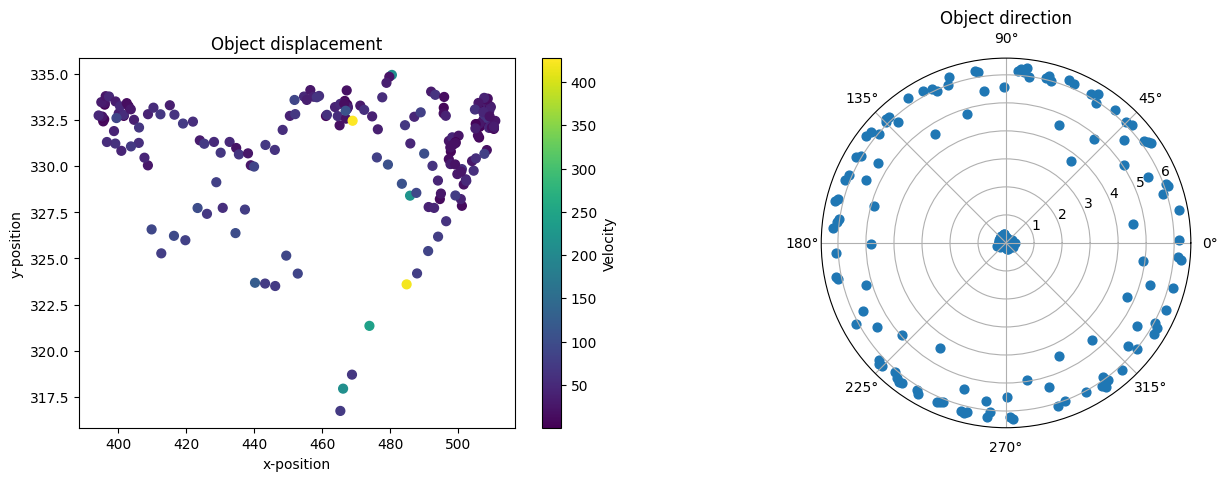# Data analysis using Julia

Permission is granted to copy, distribute and/or modify this document.This program is distributed in the hope that it will be useful, but WITHOUT ANY WARRANTY; without even the implied warranty of MERCHANTABILITY or FITNESS FOR A PARTICULAR PURPOSE.

## Data analysis using Julia#

### Introduction#

The Julia documentation and installation guide can be found at https://julialang.org/. We provide here a simple example that details how to import the `tracking.txt` file from FastTrack, and how to extract basic information like the number of objects, the number of images etc...

using DataFrames
using CSV
using PyPlot
using Plots
using StatsPlots

### Importation#

We are going to import the tracking file in a DataFrames. Note that the user needs to provide the full path to the `tracking.txt` file.

display(data)

2,475 rows × 23 columns (omitted printing of 14 columns)

Float64Float64Float64Float64Float64Float64Float64Float64Float64
1514.327333.125.81619499.96327.7276.10226508.345330.8765.94395
2463.603327.0510.301279449.585330.3230.245547458.058328.3460.238877
323.9978287.7153.7064634.9722278.8363.9981929.2056283.5053.84844
4372.536230.1430.194641354.226231.6046.08737364.822230.7590.0515087
5480.58213.4821.28236478.125228.521.53303479.428220.5431.42567
6171.682143.556.09077155.507140.1166.1146164.913142.1136.08216
7498.151121.326.00177483.712119.2850.0223247492.683120.556.15298
8329.56123.4186.08726312.526119.0425.9098322.531121.6146.01722
9465.256115.0454.44359470.05799.9114.40559467.106109.2054.40862
10423.66366.37890.0888056409.10567.29716.12053417.61566.76230.0292602
11424.48740.42325.48198411.59430.39125.88869418.9636.11925.64923
12370.59135.21475.99688354.67229.56335.89121364.00732.87675.94008
13498.50220.25275.66339487.2549.194995.39497493.75815.57815.5026
14367.7915.030346.05933352.0766.756030.653641361.125.759040.152688
15512.965332.5755.86617499.435327.7596.052507.626330.6735.95102
16463.385324.6590.707451.431332.1930.246265458.959327.4430.542368
1719.4579293.0224.2886125.5579281.2064.1837921.8962288.3024.23379
18379.037230.5276.10571361.728229.6160.199343371.74230.1446.25939
19478.884206.7121.27832475.454221.7571.40929477.197214.1081.35472
20173.923143.0420.00732468157.261142.1826.00453167.066142.6896.20403
21498.561122.6875.83253486.357118.1966.13893493.718120.9065.95151
22328.812124.1346.05932312.848119.6055.98617322.331122.2946.00901
23461.738116.7314.47649466.371101.7364.40285463.615110.6564.41641
24428.63169.27155.87139415.66564.64446.13862423.21867.33645.96558
25425.82144.99425.59983414.8433.20285.37159421.24840.08975.461
26368.36235.62195.97427353.2230.46255.88261362.10933.48915.94605
27503.48422.72935.76026489.63216.63155.92136497.92420.28575.86668
28369.1845.840746.15994352.6224.253286.24787362.1445.167666.19236
29510.519331.4175.88883495.784327.3666.12889504.484329.7586.02088
30464.242323.5330.290639451.756328.1940.532686459.432325.3260.37736
&vellip;&vellip;&vellip;&vellip;&vellip;&vellip;&vellip;&vellip;&vellip;&vellip;

### Basic information#

We are going to extract the basic tracking information:

• Object's id
• Number of objects
• Number of images
• Number of images with at least one object detected
objects = Set(data.id)
print("Objects id: ", objects)
Objects id: Set([10, 5, 9, 8, 13, 4, 1, 0, 12, 7, 11, 2, 3, 6])
numObjects = length(objects)
print("Number of objects: ", numObjects)
Number of objects: 14
numImages = maximum(data.imageNumber) + 1 # Image index starting at 0
print("Number of images: ", numImages)
Number of images: 200
numDetected = length(Set(data.imageNumber))
print("Number of images with at least one object detected: ", numDetected)
Number of images with at least one object detected: 200

### Basic plots#

We are going to make basic plots using Plots, StatsPlots and the PyPlot (that require a valid matplotlib installation) modules. For more information about plotting see https://docs.juliaplots.org/latest/tutorial/.

objectsByImage = zeros(numImages)
for i in 1:numImages
objectsByImage[i] = length(Set(data.id[data.imageNumber .== i-1]))
end
Plots.plot(1:numImages, objectsByImage; title="Number of detected objects by frame", xlabel="Frames", ylabel="Objects", label=false)dataObject0 = data[data.id .== 0, :]
distance = sqrt.(diff(dataObject0.xBody).^2 + diff(dataObject0.yBody).^2)
framerate = 25
time = diff(dataObject0.imageNumber)/framerate
velocity = distance./time
fig, ax = PyPlot.subplots(1, 2)
ax = PyPlot.subplot(121)
plot = ax.scatter(dataObject0.xBody[1:end-1], dataObject0.yBody[1:end-1], c=velocity, s=40)
ax.set_title("Object displacement")
ax.set_xlabel("x-position")
ax.set_ylabel("y-position")
bar = fig.colorbar(plot)
bar.set_label("Velocity")
ax = PyPlot.subplot(122, projection="polar")
ax.scatter(1:length(dataObject0.tBody), dataObject0.tBody, s=40)
ax.set_title("Object direction")PyObject Text(0.5, 1.0715488215488216, 'Object direction')
velocities = Any[]
for i in 1:numObjects
distance = sqrt.(diff(data.xBody[data.id .== i-1]).^2 + diff(data.yBody[data.id .== i-1]).^2)
time = diff(data.imageNumber[data.id .== i-1])/25
velocity = distance./time
append!(velocities, [velocity])
end
StatsPlots.boxplot(velocities, label=false, title="Velocity distributions", ylabel="Velocity (px/s)", xlabel="Objects")